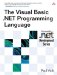# Floating-Point Data Types

 <  Day Day Up  >

The floating-point data types store numbers that can have fractional parts, and are most useful when you are dealing with values that are not integers. A floating-point number has three parts : a whole part (the part to the left of the decimal point), a fractional part (the part to the right of the decimal point) called the mantissa, and an exponent . The exponent indicates the power of ten to multiply the other two parts by to get the actual value of the floating-point number. For example, the floating-point literal 1.5E3 is equivalent to the floating-point literal 1500.0 . While the floating-point types can represent a large range (such as 1E+308), they have limited precision. This means that a sufficiently large or small value can keep only a certain number of significant digits. The Double type can preserve approximately 18 digits of precision, while the Single type can preserve only about 8 digits of precision. The default value of the floating-point types is the literal 0.0 .

## Style

The Double type is the more natural floating-point size on the .NET Framework ”the Single type should not be used unless explicitly needed.

The exact behaviors of the floating-point types are defined by the .NET Framework and are beyond the scope of this book. However, a few pitfalls are worth mentioning. Floating-point values differ from integer values in that they have special values such as positive and negative zero, positive and negative infinity, and NaN (Not a Number). This last value is the most interesting because the result of dividing a floating-point number by zero is NaN instead of an exception. In the following example, the code will run without error even though there is division by zero, and the variable a will end up with the value of NaN.

` Dim a, b As Double a = 1.5 b = 0.0 a = a / b `

## Compatibility

In previous versions of Visual Basic, the runtime environment would throw an exception if the result of a floating-point operation was NaN. This is no longer the case, so Visual Basic .NET programmers must always check the result of floating-point division to ensure that it is not NaN. The functions Double.IsNaN and Single.IsNaN can be used for this purpose.

Another thing to be aware of is that the .NET Framework may calculate floating-point operations at whatever precision the machine that the code is running on can support, and this may be a higher precision than Double or Single supports. As a result, comparing the results of floating-point operations may give unexpected results. Take the following example.

` Dim a, b As Double a = 1.0 / 3.0 b = 1.0 / 3.0 If a = b Then   Console.WriteLine("equal") Else   Console.WriteLine("not equal") End If `

Depending on how the code is compiled by the JIT compiler at runtime, either result may be printed because one or both of the division operations may be calculated at a higher precision than the other.

#### Floating-Point Literals

Floating-point literals have same three parts as a floating-point value: a whole part, a mantissa, and an exponent. The whole part is just an integer literal and is followed by a decimal point. The decimal point is followed by the mantissa represented as an integer literal. An exponent can be specified by adding the character E to the end and then a signed integer literal specifying the exponent. The following shows some examples of floating-point literals.

` Dim a As Single Dim b, c, d As Double a = 1.5F b = 3E-30 c = 4R d = 0.4 `

If a floating-point value specifies an exponent, it can choose to omit the mantissa. The type of a floating-point literal is always Double , unless it is explicitly typed using a type character: F for Single and R for Double .

## Design

The choice of characters is somewhat strange because of a lack of suitable characters-S is already taken for Short and D is already taken for Decimal . F was chosen for Single because the type has historically been referred to as "float," while R was chosen for Double because the type has historically been referred to as "real."

An integer literal can also be turned into a floating-point literal by adding the floating-point type characters.

## Style

The characters ! and # can be used as type characters for Single and Double , respectively, but their inclusion is for historical reasons, and their use is discouraged.

 <  Day Day Up  >The Visual Basic .NET Programming Language
ISBN: 0321169514
EAN: 2147483647
Year: 2004
Pages: 173
Authors: Paul Vick

Similar book on Amazon